# Area of rectangles and the distributive property | Measurement | Pre-Algebra | Khan Academy

##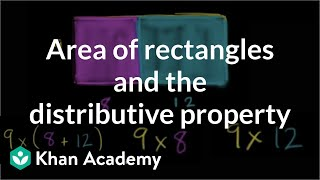By Khan Academy

Use the distributive property to find area of rectangles# Area of rectangles and rectangular shapes. Concrete case of the distributive property (3.MD.7.c)

##By Math Mammoth

Area of rectangles can be found by multiplication. In my example rectangle, we count the rows and columns, and then write a multiplication to find the area (5 x 3 = 15 squares).# Area of rectangles and rectangular shapes. Concrete case of the distributive property (3.MD.7.c)

##By Math Mammoth

the area of a rectangle with whole-number side lengths a and b + c is the sum of a × b and a × c.# Area of diagonal generated triangles of rectangle are equal | Geometry | Khan Academy

##By Khan Academy

In this video, Mr. Khan draws a box and two intersecting lines that produce several triangles. Mr. Khan proves that the area of these diagonal-generated triangles of the rectangle are equal. He goes over the formula for finding the area of a triangle. Sal Khan is the recipient of the 2009 Microsoft Tech Award in Education. The screen gets busy (and dark)--the viewer may want to open to 'full screen' to see everything. The sound is a little low# Rectangle area as product of dimensions same as counting unit squares | Pre-Algebra

##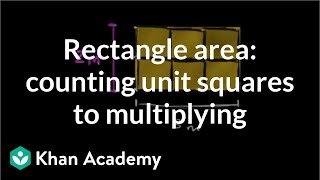By Khan Academy

Use unit squares to see why multiplying side-lengths can also find the area of rectangles. ï¿½ï¿½ï¿½ï¿½ï¿½ï¿½ï¿½ï¿½ï¿½ï¿½ï¿½ï¿½ï¿½ï¿½ï¿½ï¿½ï¿½ï¿½ï¿½ï¿½ï¿½ï¿½ï¿½ï¿½# Rectangle area as product of dimensions same as counting unit squares

##By Khan Academy

Use unit squares to see why multiplying side-lengths can also find the area of rectangles. ï¿½ï¿½ï¿½ï¿½ï¿½ï¿½ï¿½ï¿½ï¿½ï¿½ï¿½ï¿½ï¿½ï¿½ï¿½ï¿½ï¿½ï¿½ï¿½ï¿½ï¿½ï¿½ï¿½ï¿½# Transitioning from counting to multiplying to find area | 3rd grade | Khan Academy

##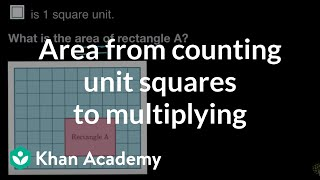By Khan Academy

finds the area of a rectangle both by counting unit squares and multiplying side lengths# [3.MD.7d-2.0] Adding Partial Areas - Common Core Standard

##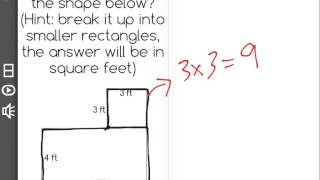By Freckle education

Find areas of rectilinear figures by decomposing them into non-overlapping rectangles and adding the areas of the non-overlapping parts.# [3.MD.7b-1.0] Multiply to find Area - Common Core Standard

##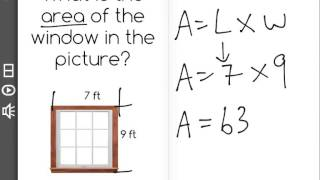By Freckle education

Multiply side lengths to find areas of rectangles with whole-number side lengths in the context of solving real world and mathematical problems# Measuring the same rectangle with different unit squares

##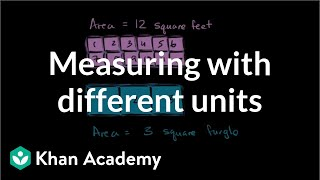By Khan Academy

Find area of a rectangle with different sized units.# Comparing areas of plots of land | Measurement | Pre-Algebra

##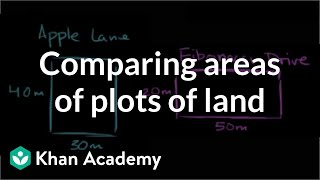By Khan Academy

Find area of two rectangles to solve a word problem.# Area of a Rectangle | MathHelp.com

##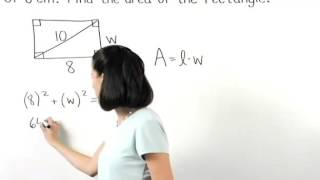By MathHelp.com# Finding area by multiplying

##By Khan Academy

Find area of a rectangle with different sized units.# Finding area by multiplying

##By Khan Academy

Find area of a rectangle with different sized units.# Finding area by multiplying

##By Khan Academy

Find area of a rectangle with different sized units.# Finding area by multiplying

##By Khan Academy

Find area of a rectangle with different sized units.# Area for Kids

##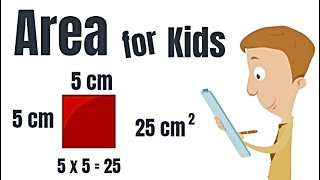By

Math is fun! Learn about area in this math video for kids! You will learn the difference between perimeter and area, learn how area works and even learn how to find the area of rectangles and squares!# Multiply monomials by monomials

##By Khan Academy

Sal expresses the area of a rectangle with length 4xy and width 2y as a monomial.# Multiply monomials by monomials

##By Khan Academy

Sal expresses the area of a rectangle with length 4y and width 2y as a monomial.# Finding Area

##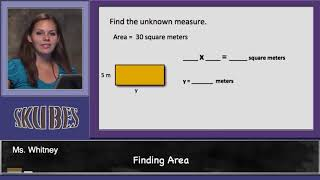By Skubes ed

Apply the area and perimeter formulas for rectangles in real world and mathematical problems